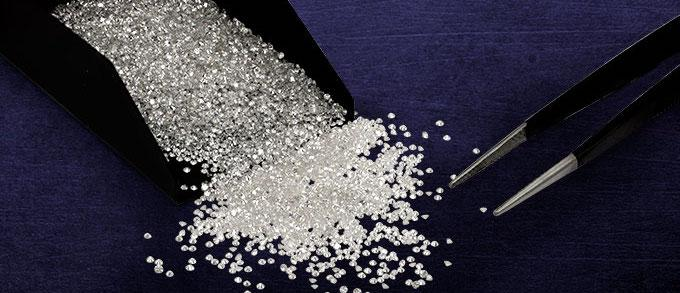in Jewelry Blog

# How to Calculate a Diamond’s Carat Weight

You can calculate your diamond’s carat value by measuring it with a ruler that has millimeters on it. That’s the standard measurement for jewelry. Or you can weigh the stone if you have an accurate scale calibrated in grams and milligrams.

#### Definition of Carat

A carat is a measure of weight. It is exactly 200 milligrams, or .2 grams (about as heavy as a paper clip). Because it’s a measure of weight, and not of volume or size, no two carat-weighted gems will be exactly the same size. One will almost certainly have a somewhat greater density than the other. This is especially true of different types of gemstones, such as rubies, emeralds or diamonds.

For this to work, you must have a very accurate scale and separate the diamond from its mounting. Weigh just the gemstone and record its weight in grams. Then divide that number by .2 to obtain the carat-weight. So, for example, if your diamond weighs .3 grams, then dividing that number by .2 would yield 1.5, or 1 ½ carats. If you don’t have access to such a scale, don’t despair. You can simply measure your diamond.

All you need for this measurement is a small ruler calibrated in millimeters. If your diamond is round, simply measure the diameter of the stone, looking down on it. Let’s say it’s six millimeters. Then you measure the depth of the stone. That’s the length from the top of the stone to its pointy bottom. Let’s say it’s three millimeters.

Now multiply 6 x 6 (diameter squared) x 3 x .006. The result is .648, or about .65 of a carat. Keep in mind that this will be an approximate value.

If you have an oval ring, this will work. Just multiply the millimeters across one length of the oval and then the other one. Let’s say it’s 6 mm. one way and 10 mm. the long way. Just take 6 plus 10 (16) and divide by 2 to obtain its diameter (8). Then you measure the depth the same way you do for a round stone, and multiply by the same co-efficient. The depth measures 4 mm. Thus you have 8 x 8 x 4 x .006 = 1.536 carats.

This measurement won’t be exact, but will give you some idea.

#### Measuring Other Cuts

If your diamond isn’t round or oval, you will have different measurements to make and different coefficients to multiply by, but the process is roughly the same.

This process will work on radiant, Asscher, square and rectangular cuts. Here’s the formula:

Length x width x depth x Coefficient

Here are the coefficients you would use for different cuts. A coefficient is simply the ratio of length to width.

L/W ratio 1.25: .008

L/W ratio 1.50: .009

L/W ratio 2:00: .01

L/W ratio 2:50: .0105

You may have a stone that is in between these values, so you can use the closest one.

Again, these measurements will give you an approximate idea of the carat value of your diamond. It will not be exact, but the knowledge will be reassuring.